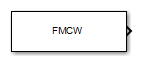# FMCW Waveform

Frequency-modulated continuous (FMCW) waveform source

•Libraries:
Phased Array System Toolbox / Waveforms

## Description

The FMCW Waveform block generates a frequency modulated continuous wave (FMCW) waveform with a specified sweep time and sweep bandwidth. The block output can be either an integer number of pulses or samples.

## Ports

### Output

expand all

FMCW waveform, returned as a complex-valued column vector containing the waveform samples.

• If the Output signal format parameter is set to `Samples`, then the output of the port consists of the number of samples set by the Number of samples in output parameter.

• If the Output signal format parameter is set to `Sweeps`, then the output of the port consists of the number of sweeps set by the Number of sweeps in output parameter. Also, if the Sweep direction parameter is set to `Triangle` each sweep is one-half period in duration.

## Parameters

expand all

Sample rate of the output waveform, specified as a positive scalar. The ratio of Sample rate (Hz) to each element in the Pulse repetition frequency (Hz) vector must be an integer. This restriction is equivalent to requiring that the pulse repetition interval is an integral multiple of the sample interval.

#### Programmatic Use

 Block Parameter:`SampleRate` Type:double Values:positive scalar Default:`1e6`

Specify the duration, in seconds, of the upsweep or the downsweep of the signal as a scalar or row vector of positive, real numbers. The product of the Sample rate value and each Sweep time entry must be an integer.

To implement a varying sweep time, specify Sweep time as a row vector. The waveform uses successive entries of the vector as the sweep time for successive periods of the waveform. If the last element of the vector is reached, the process continues cyclically with the first entry of the vector.

If Sweep time and Sweep bandwidth are both row vectors, the vectors must have the same length.

If Sweep direction is `Up` or `Down`, the sweep period equals the sweep time. If Sweep direction is `Triangle`, the sweep period is twice the sweep time because each period consists of an upsweep segment and a downsweep segment.

Specify the bandwidth of the linear FM sweeping, in hertz, as a scalar or row vector of positive, real numbers.

To implement a varying bandwidth, specify Sweep bandwidth as a row vector. The waveform uses successive entries of the vector as the sweep bandwidth for successive periods of the waveform. If the waveform reaches the last element of the Sweep bandwidth vector, the process continues cyclically with the first entry of the vector.

If Sweep time and Sweep bandwidth are both row vectors, the vectors must have the same length.

Specify the direction of the linear FM sweep as one of `Up`, `Down`, or `Triangle`.

If you set this parameter value to `Positive`, the waveform sweeps in the interval between 0 and B, where B is the value of the Sweep bandwidth parameter. If you set this parameter to `Symmetric`, the waveform sweeps in the interval between –B/2 and B/2.

Specify the format of the output signal as `Sweeps` or `Samples`.

If you set this parameter to `Sweeps`, the output of the block is in the form of multiple sweeps. The number of sweeps is the value of the Number of sweeps in output parameter.

If you set this parameter to `Samples`, the output of the block is in the form of multiple samples. The number of samples is the value of the Number of samples in output parameter.

If the Sweep direction parameter is set to `Triangle`, each sweep is one-half of a period.

Number of samples in the block output, specified as a positive integer.

Example: `1000`

#### Dependencies

To enable this parameter, set the Output signal format parameter to `Samples`.

#### Programmatic Use

 Block Parameter:`NumSamples` Type:double Values:```positive scalar``` Default:`100`

Data Types: `double`

Specify the number of sweeps in the block output as a positive integer. This parameter appears only when you set Output signal format to `Sweeps`.

Block simulation, specified as `Interpreted Execution` or `Code Generation`. If you want your block to use the MATLAB® interpreter, choose `Interpreted Execution`. If you want your block to run as compiled code, choose ```Code Generation```. Compiled code requires time to compile but usually runs faster.

Interpreted execution is useful when you are developing and tuning a model. The block runs the underlying System object™ in MATLAB. You can change and execute your model quickly. When you are satisfied with your results, you can then run the block using ```Code Generation```. Long simulations run faster with generated code than in interpreted execution. You can run repeated executions without recompiling, but if you change any block parameters, then the block automatically recompiles before execution.

This table shows how the Simulate using parameter affects the overall simulation behavior.

When the Simulink® model is in `Accelerator` mode, the block mode specified using Simulate using overrides the simulation mode.

Acceleration Modes

 Block Simulation Simulation Behavior `Normal` `Accelerator` `Rapid Accelerator` `Interpreted Execution` The block executes using the MATLAB interpreter. The block executes using the MATLAB interpreter. Creates a standalone executable from the model. `Code Generation` The block is compiled. All blocks in the model are compiled.

 Block Parameter:`SimulateUsing` Type:enum Values:```Interpreted Execution```, `Code Generation` Default:```Interpreted Execution```